﻿ Fluidized bed (Laminar) - XSim

# Fluidized bed (Laminar)

Update: July 28, 2017
OpenFOAM 4.x

$FOAM_TUTORIALS/multiphase/twoPhaseEulerFoam/laminar/fluidisedBed ## Summary We calculate the air flow blowing in from the bottom of air-mixed sand for 2 seconds. The calculation is performed as a two-dimensional problem with one mesh in the z-direction.Model geometry The physical properties of air and particles are set in the files "constant/phaseProperties", "constant/thermophysicalProperties.air", "constant/thermophysicalProperties.particles". The turbulence settings for air and particles are set in the files "constant/turbulenceProperties.air" and "constant/turbulenceProperties.particles". Laminar flow (direct calculation) is used for the air, and a "particle-particle" pressure model is used for the particles. In this model, we calculates the pressure "p" of the phase from the volume ratio "α" based on the following equation.In default configuration, pα=500、 emax=1000、αmax＝=0.62、g0=1000. The meshes are as follows, and the number of mesh is 6000.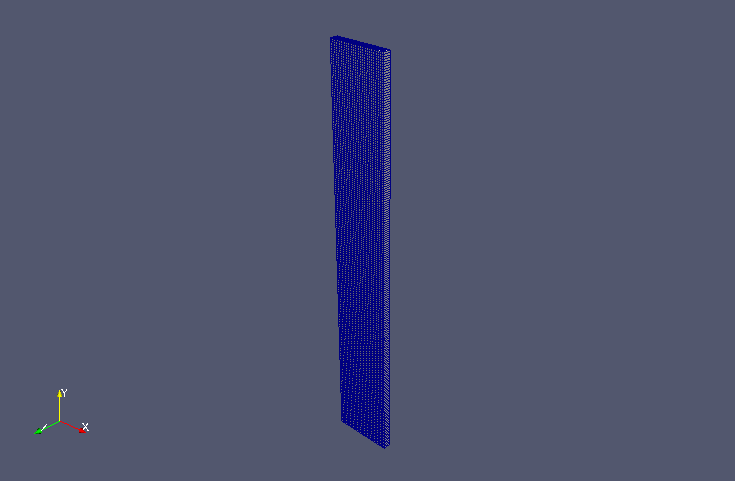Meshes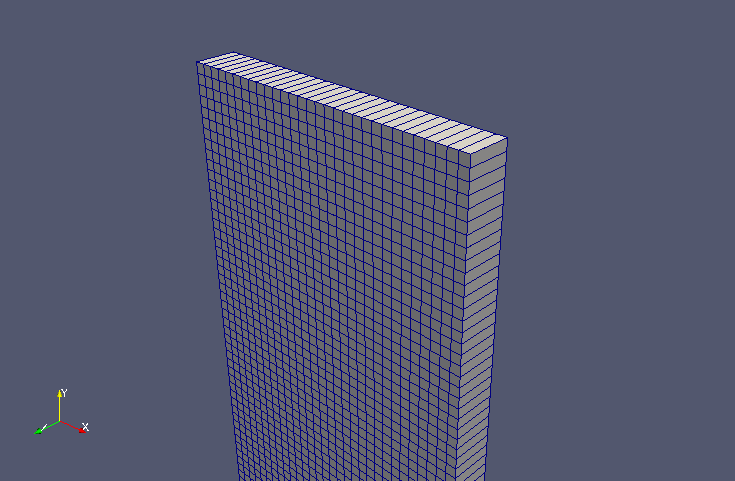Meshes (zoomed) The calculation result is as follows. Volume ratio of particles (alpha.particles)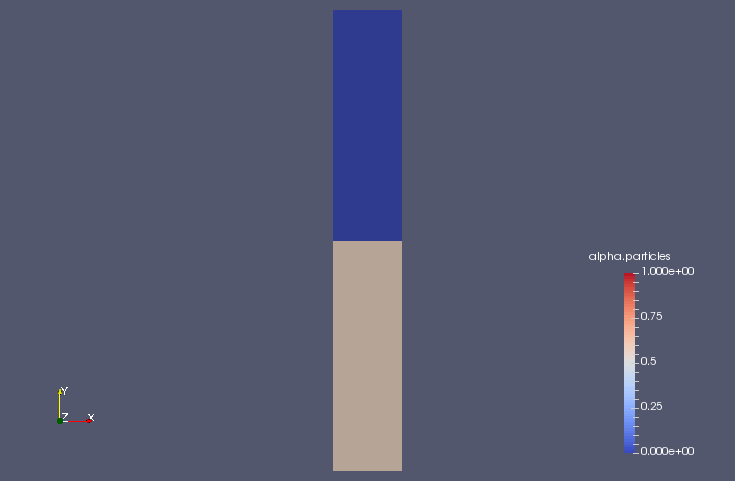Volume ratio of particles at initial time (alpha.particles)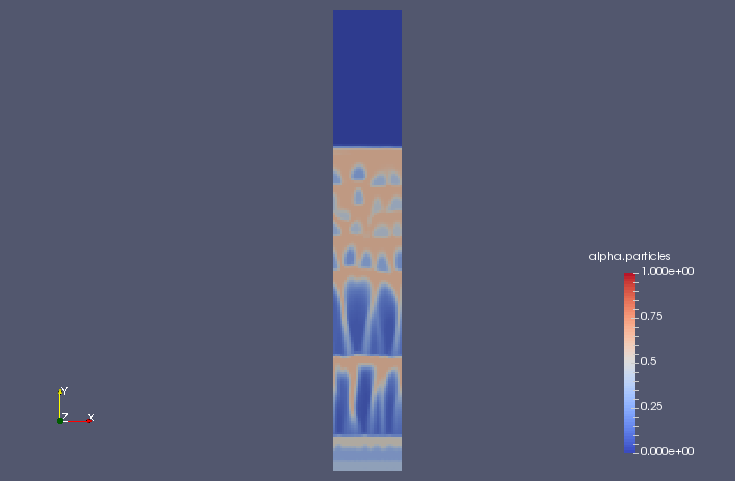Volume ratio of particles at 0.6 sec (alpha.particles)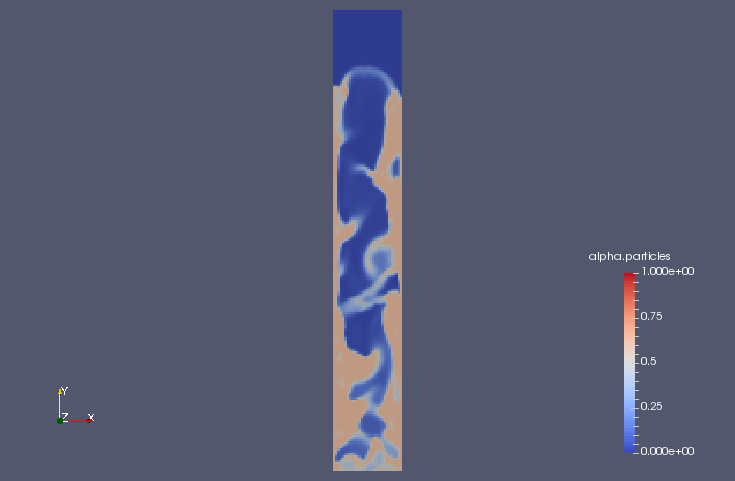Volume ratio of particles at 1 sec (alpha.particles) ## Commands cp -r$FOAM_TUTORIALS/multiphase/twoPhaseEulerFoam/laminar/fluidisedBed fluidisedBed
cd fluidisedBed

blockMesh
setFields

twoPhaseEulerFoam

paraFoam

## Calculation time

18 minutes 16.93 seconds *Single, Inter(R) Core(TM) i7-2600 CPU @ 3.40GHz 3.40GHz